General Overview of TRS-80 Graphics
 The TRS-80 had very rudimentary graphics. Little rectangles could be displayed to a maximum of 128 squots (square dots) across by 48 down, always beginning with row 0. Squots could be put onto the screen with the SET(X,Y) command, removed with the RESET(X, Y) command, and checked for on/off status with the POINT (X, Y) command. However, using SET/RESET was the slowest way to manipulate graphics and to do anything approximating gaming one would need another method. In addition to the SET/RESET to control each squot,the TRS-80 had a character set which would include a 2 x 3 squot matrix. These characters, from 128 through 191, ran the gamut from completely off/black (128) to completely on (191), and everything in between. The 2 x 3 matrix running from 128 to 191 was actually an 8-bit binary representation of a 7 bit number “1xxxxxxx” so if you wanted the top two squots it would be “10000011”, the middle 2 squots would be “10001100”, etc. The actual CHR\$(xx) representations appear below on this page. But there’s more!! While you could use PRINT and PRINT@ to put those CHR\$(xxx) matrices on the screen (putting up 6 squots at once), you could also pack them into strings and move those strings around. This is called “String Packing” and is virtually identical to the packing technique used in embedding machine language routines in BASIC programs. String Packing is explained below. Once run, the routine is called by a simple X=USR(0) command. Choosing the former will allow you to save a program with the string embedded, and then you would just need lines 10, 30 and 50 in the future.

TRS-80 Graphic Worksheet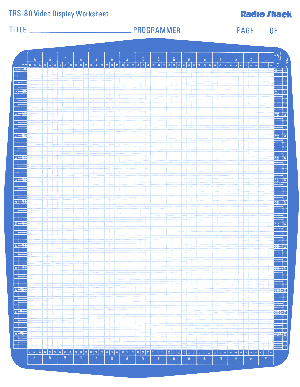Can be downloaded here

Graphic Characters
 128 =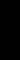129 =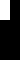130 =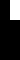131 =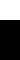132 =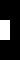133 =134 =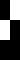135 =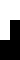136 =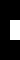137 =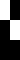138 =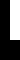139 =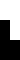140 =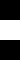141 =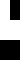142 =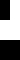143 =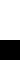144 =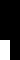145 =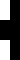146 =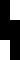147 =148 =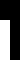149 =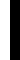150 =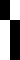151 =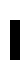152 =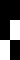153 =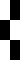154 =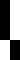155 =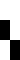156 =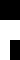157 =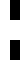158 =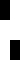159 =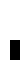160 =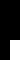161 =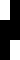162 =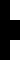163 =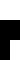164 =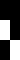165 =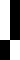166 =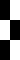167 =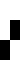168 =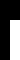169 =170 =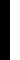171 =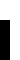172 =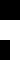173 =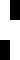174 =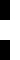175 =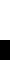176 =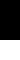177 =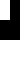178 =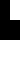179 =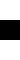180 =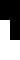181 =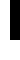182 =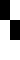183 =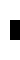184 =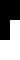185 =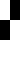186 =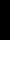187 =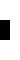188 =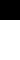189 =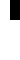190 =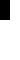191 =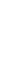NOTE: Since the TRS-80 screen is black, each above square represents what the screen would look like with that character on it. For example, 128 shows nothing and 191 shows a completely filled in block.

Graphic String Packing
 The general way to pack a string would be: 10 X\$=”///////////////////////” : REM Number of Slashes to Correspond to # of Characters 20 A = PEEK(VARPTR(X\$)+1) : B = PEEK(VARPTR(X\$)+2) : C = A + 256 * B 30 FOR D = C TO C+LEN(X\$) 40 INPUT E 50 POKE D, E 60 NEXT T Of course, you could feed the routine with DATA statements as well, but the result would be that X\$ would be transformed to a string looking like “RESETSETCLSPRINT….” but when printed, it would show the graphics you had set up.

1.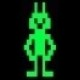Luc Gevaert